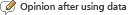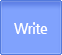연구성과물검색
유형별/분류별 연구성과물 검색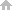HOME > Search by Achievements Type > Articles View

#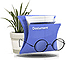Articles Detailed Information

Valuation Ideals of Order Two in 2-dimensional Regular Local Rings
Article NRF is supported by Research Projects( 학습조직의 강화와 학교재건을 위한 교육과정/수업/평가/테크놀로지 통합 체제 연구 및 개발: 중등 국어, 수학 교과를 중심으로 | 2001 Year 중점연구소지원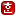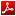| 노선숙(이화여자대학교)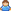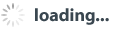) data is submitted to the '연구성과물'
• This is the information that experts who participate in DB construction project of KRM have added input .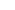ᅟPublication History / pp. 1 ~ 17 Primary Author 노선숙 (이화여자대학교) Index terms valuation, valuation ideal, value semigroup, order of ideal, rank of valuation, simple valuation ideal
•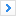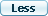Abstract(Main language)
• Let K be the quotient field of a 2-dimensional regular local ring (R;m) and let v be a prime divisor of R, i.e., a valuation of K birationally dominating R which is residually transcendental over R. Zariski showed that: such prime divisor v is uniquely associated to a simple m-primary integrally closed ideal I of R, there are only finitely many simple v-ideals including I, and all the other v-ideals can be uniquely factored into products of simple v-ideals. The number of nonmaximal simple v-ideals is called the rank of v or the rank of I as well. It is also known that such anm-primary ideal I is minimally generated by o(I)+1 elements, where o(I) denotes the m-adic order of I. Given a simple valuation ideal of order two associated to a prime divisor v of arbitrary rank, in this paper we find minimal generating sets of all the simple v-ideals and the value semigroup v(R) in terms of its rank and the v-value difference of two elements in a regular system of parameters of R. We also obtain unique factorizations of all the composite v-ideals and describe the complete sequence of v-ideals as explicitly as possible.
•TOC
• Ⅰ. INTRODUCTION
Ⅱ. Valuation ideals of small rank
Ⅲ. b = odd case
Ⅳ. b = even case
Ⅴ. Sequence of v-ideals
REFERENCES
•List of digital content of this articles• This document, it is necessary to display the original author and you do not have permission